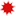SEARCH Advanced Search Topic All Topics Algebra Applied Mathematics Calculus and Analysis Chemistry Computer Science Courseware Differential Equations Discrete Mathematics Earth Sciences Economics and Finance Engineering Geometry Graphics Life Sciences Modeling and Simulation Number Theory Physics Probability and Statistics Programming Recreational Social Sciences Tutorial and Reference Language All Languages English Bulgarian Catalan Chinese Dutch Finnish French German Greek Hungarian Italian Japanese Korean Lithuanian Norwegian Polish Portuguese Russian Spanish Swedish
 BROWSE TOPICS Algebra» Applied Mathematics» Calculus and Analysis» Chemistry» Computer Science» Courseware» Differential Equations» Discrete Mathematics» Earth Sciences» Economics and Finance» Engineering» Geometry» Graphics» Life Sciences» Modeling and Simulation» Number Theory» Physics» Probability and Statistics» Programming» Recreational» Social Sciences» Tutorial and Reference»VisualDSolve, Second Edition
by Antonin Slavik, Stan Wagon, Dan Schwalbe
• Year: 2018
• ISBN: 9780965053273 (ebook)
•Based on latest Wolfram Language version
Description
VisualDSolve is a Mathematica e-book and accompanying package showing how Mathematica's visualization tools can be used to enhance the viewing of solutions to differential equations. The book contains 18 chapters; the first 5 serve as a manual to the many functions of the package, and the other 13 are comprehensive examples. The package itself has some new ways of viewing differential equations. Two noteworthy examples are the use of curvy fish shapes to describe the vector fields determined by a differential equation, and the use of colored nullcline regions to describe the different types of directions for an autonomous system of two equations.

Note: VisualDSolve is available as a download only. It is compatible with Mathematica 10 or greater. Contents
VisualDSolve | Auxiliary Functions | SystemSolutionPlot | PhasePlot | SecondOrderPlot | Differential Equations and Mathematica | Some Parachute Experiments | Linear Systems | Logistic Models of Population Growth | Hamiltonian Systems | A Devilish Equation | Lead Flow in the Human Body | Making a Discus Fly | A Double Pendulum | The Duffing Equation | The Tetrapods of Wada | I Tossed a Book into the Air | Miscellany Related Topics
Courseware, Differential Equations